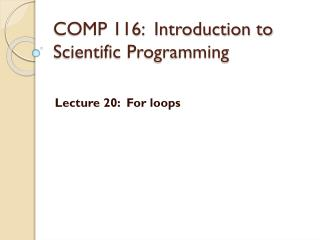Download PresentationCOMP 116: Introduction to Scientific Programming

# COMP 116: Introduction to Scientific Programming

Download Presentation## COMP 116: Introduction to Scientific Programming

- - - - - - - - - - - - - - - - - - - - - - - - - - - E N D - - - - - - - - - - - - - - - - - - - - - - - - - - -
##### Presentation Transcript

1. COMP 116: Introduction to Scientific Programming Lecture 20: For loops

2. The counted loop solution: for loops forvar= vector commands end Keywords • Read this as: • Run commands as many times as there are entries in vector • Each time, assign var to be equal to one of the entries

3. A=[2 8 3 12]; fori=1:length(A) A(i)=A(i)+1; disp(A(i)); end A=[2 8 3 12]; A(1)=A(1)+1; disp(A(1)); A(2)=A(2)+1; disp(A(2)); A(3)=A(3)+1; disp(A(3)); A(4)=A(4)+1; disp(A(4)); Output: 3 9 4 13

4. What does this code do? % Assume A is a preassigned vector var=0; fori=1:length(A) var=var+A(i); end

5. What does this code do? % Assume that A is a preassigned vector B=zeros(size(A)); for i=1:length(A) B(i)=A(i)*A(i)+A(i)-1 end

6. Functions exercise • Write a function that • Given an input x • Returns an output x^2+x-1

7. Using functions • Problem: Plot x^2+x-1 vs x(without using element-wise multiplication or exponentiation) • Plan: • Create a vector A of 1000 uniformly spaced numbers between -5 and 5 • Use the function we have just written in a for loop to create the vector B, such that B(i)=A(i)*A(i)+A(i)-1 • Plot B vs A.

8. Assignment 2 • plot returns a handle that can be used to modify the figure later. • >>h=plot(x,y); % h is the handle here. • >>set(h, ’Color’, ’r’); % changes the color to red • Write a script that • Plots N squares at random centers with random edge-lengths • Then allows the user to repeatedly click on the figure window (using ginput). • The squares that contains the clicked point are colored red.